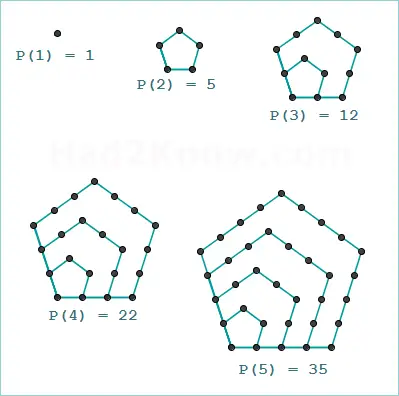# Pentagonal Number Calculator

Pentagonal Number Calculator
n =

nth pentagonal number
sum of first n pentagonal numbers
nth generalized pentagonal number
sum of first n generalized pentagonal
numbers

Pentagonal numbers are figurate numbers that arise from generalizing the concept of triangular numbers and square numbers. The figure below shows how pentagonal numbers are related to nested pentagons whose side length increases by a constant amount. The first ten pentagonal numbers are 1, 5, 12, 22, 35, 51, 70, 92, 117, and 145. The nth pentagonal number P(n) is given by

P(n) = n(3n-1)/2

If you allow n to be 0 or negative, you can extend the sequence to obtain the generalized pentagonal numbers. Excluding 0, the first 20 generalized pentagonal numbers are

1, 2, 5, 7, 12, 15, 22, 26, 35, 40, 51, 57, 70, 77, 92, 100, 117, 126, 145, and 155.

The pentagonal and generalized pentagonal numbers exhibit a number of remarkable properties. For example, every generalized pentagonal number is 1/3 of a triangular number. More properties and related formulas are given below.### Other Properties of Pentagonal Numbers

The expansion of the infinite product

G(x) = Πn=1 (1 - xn)

yields

G(x) = 1 - x - x2 + x5 + x7 - x12 - x15 + x22 + x26 - x35 - x40 + x51 + x57 - ...

The exponents in this series are the generalized pentagonal numbers.

There are infinitely many pentagonal numbers which are also square numbers. The first few are 1, 9801, 94109401, 903638458801, 8676736387298001... As you can see, this sequence grows quite rapidly. If U(n) is the nth number in this sequence, then U(n) satisfies the recursive relation

U(n+2) = 9602U(n+1) - U(n) + 200.

Likewise, there are also infinitely many pentagonal numbers which are also triangular numbers. The first few are 1, 210, 40755, 7906276, 1533776805, 297544793910, 57722156241751... This sequence also grows quite rapidly, though not as rapidly as the previous sequence. If V(n) is the nth term in the sequence, then

V(n+2) = 194V(n+1) - V(n) + 16.

To date, the only number that is known to be simultaneously triangular, square, and pentagonal is 1. It is conjectured that this is the only such number, though it has not been proven.

The sum of the reciprocals of the pentagonal numbers converges to

1/1 + 1/5 + 1/12 + 1/22 + ... = 3*ln(3) - π/√3

The sum of the reciprocals of the generalized pentagonal numbers converges to

1/1 + 1/2 + 1/5 + 1/7+ ... = 6 - 2π/√3